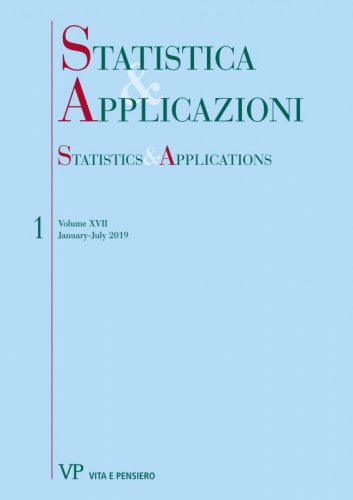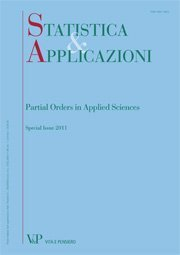Libri di Sameer A. Al-Subh - libri Statistica & Applicazioni

# Sameer A. Al-Subh

Sameer A. Al-Subh è professore presso la Jerash Private University nel dipartimento di Matematica della facoltà di Scienze in Giordania.
Share:

### Author's titles

Increasing the efficiency of ranked set sampling via visual grouping with respect to a threshold digitalYear: 2019
In this paper, a new sampling technique is proposed that carries more information than contained in ranked set sample (RSS). The proposed sampling technique is defined by making the use for the idea of visual grouping of population units with respect to a fixed threshold and RSS. We refer to it as RSS-Grouping technique. Under the best informative RSS-Grouping technique, the maximum likelihood estimator (MLE) of the mean of an exponential distribution is derived...
Estimation of the variance for logistic distribution under ranked set sampling and simple random sampling: a comparative study
Free
digitalYear: 2012
SUMMARY The logistic distribution is applicable in many area of research. In this study, several estimators of the variance when the location parameter is known and unknown are considered when data are gathered under simple random sampling (SRS) and ranked set sampling (RSS). For some estimators considered, the bias and mean square error (MSE) are not gotten in closed form. Using Monte Carlo simulations, comparison of these estimators is made based on biases, MSE and efficiency. When the estimators are compared, it is found that estimators based on maximum likelihood method are more efficient than other estimators considered, under both SRS and RSS. However, estimators based on RSS have more advantages over those based on SRS. Keywords: Logistic Distribution, Ranked set Sampling, Simple Random Sampling, Variance, Estimations.
Chi-Square goodness of fit test for logistic distribution using RSS and SRS
Free
digitalYear: 2011
In this paper, we introduce a new method to improve the power of the chi-square test for goodness of fit (GOF) of logistic distribution under selective order ranked Set sampling (RSS) based on minimum, median and maximum as compared to simple random sampling (SRS). Based on a simulation study, we illustrate that the chi-square test is found to be more powerful under selective order RSS when compared to SRS, particularly for minimum and maximum. However, for the case of median RSS, the test is found to be more powerful under SRS. To investigate these results further, the Kullback- Leibler information (KLI) is applied and similar results are found. Keywords: Goodness of fit test, chi-square test, logistic distribution, ranked set sample, simple random sample.